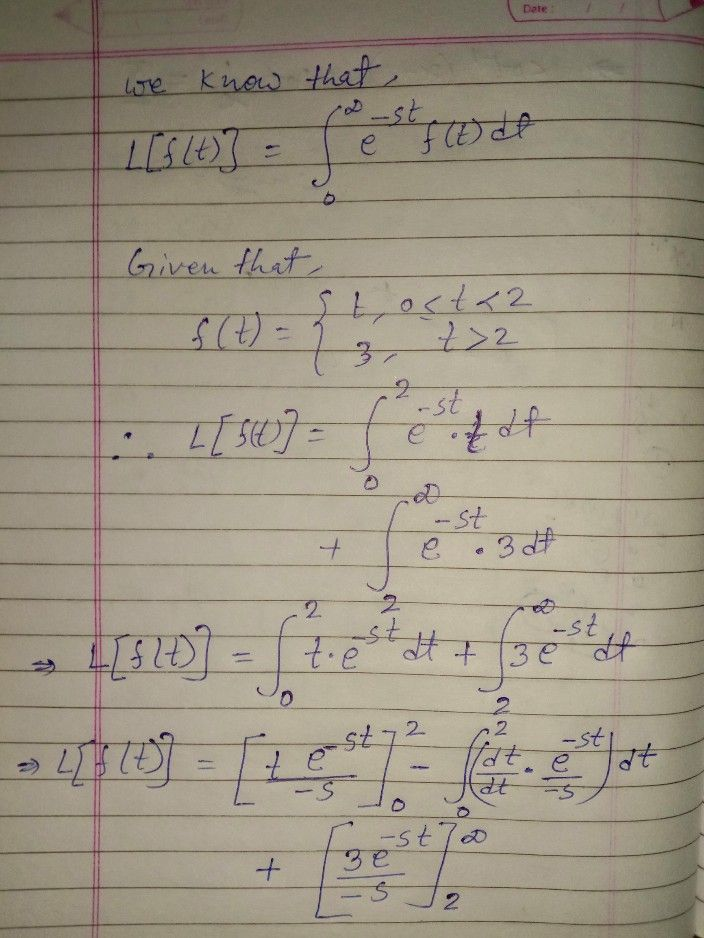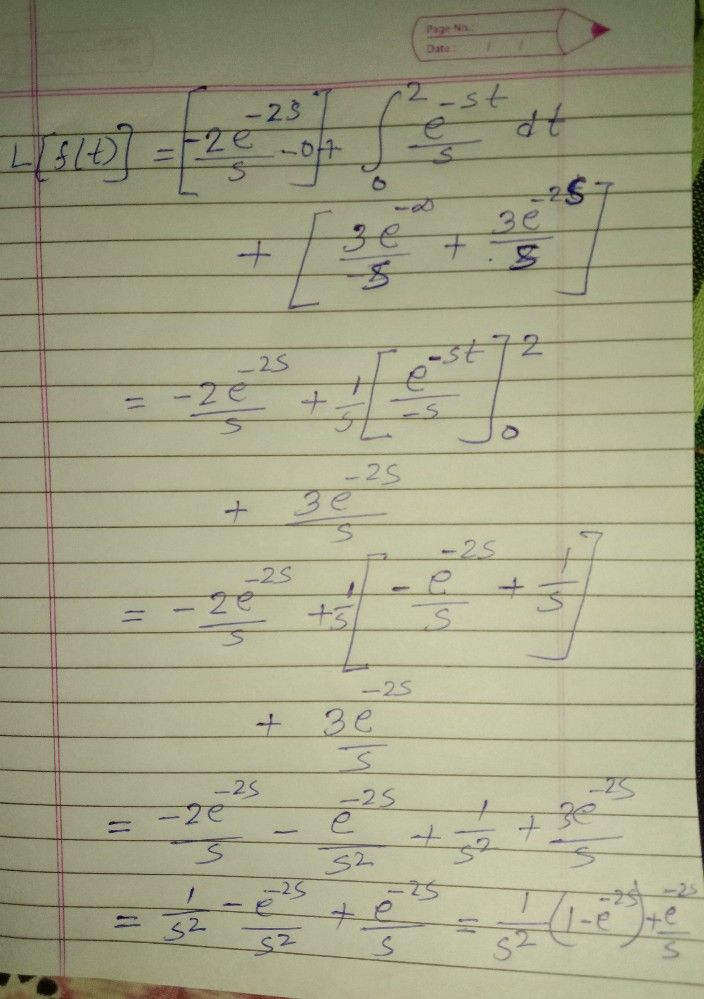Symbol
Problem$3$ $S^{-\right)}$ $3$ $5$ Find the Laplace transform of the function $f\left(t\right)= \begin{cases} t, \\ 3, \end{cases}$ $0\leq t<2$ $t>2$
10th-13th grade
Other
Search count: 141
SolutionQanda teacher - ZawedAkht